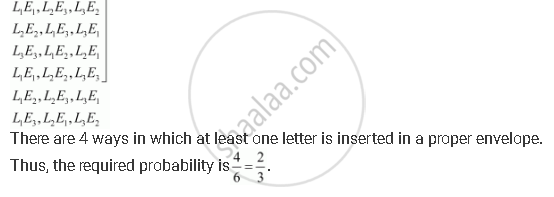CBSE (Arts) Class 11CBSE
Share

# Three Letters Are Dictated to Three Persons and an Envelope is Addressed to Each of Them, the Letters Are Inserted into the Envelopes at Random So that Each Envelope Contains Exactly One Letter. Find the Probability that at Least One Letter is in Its Proper Envelope - CBSE (Arts) Class 11 - Mathematics

ConceptProbability Probability of 'Not', 'And' and 'Or' Events

#### Question

Three letters are dictated to three persons and an envelope is addressed to each of them, the letters are inserted into the envelopes at random so that each envelope contains exactly one letter. Find the probability that at least one letter is in its proper envelope

#### Solution

Let L1L2L3 be three letters and E1E2, and E3 be their corresponding envelops respectively.

There are 6 ways of inserting 3 letters in 3 envelops. These are as follows:Is there an error in this question or solution?

#### APPEARS IN

Solution Three Letters Are Dictated to Three Persons and an Envelope is Addressed to Each of Them, the Letters Are Inserted into the Envelopes at Random So that Each Envelope Contains Exactly One Letter. Find the Probability that at Least One Letter is in Its Proper Envelope Concept: Probability - Probability of 'Not', 'And' and 'Or' Events.
S